/ +91 9829211106
Welcome to Vidhyarthi Darpan

# Maths 12th Previous Year Question Paper 2019 (CBSE)###### Maths 12th Previous Year Question Paper 2019 (CBSE)
November 30, 2019###### Maths 12th Previous Year Question Paper 2018 (CBSE)
December 2, 2019MathsSection – AQ.1. Find | AB |, if A =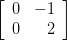and B =.

Solution:Q.2. Differentiate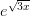, with respect to x.

Solution:

Section – BQ.6. If A = and A3 =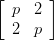| = 125, the find the value of p.

Solution:Q.12. Find the general solution of the differential equation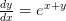Solution:

Section – CQ.21. If (a + bx)ey/x = x, then prove that

Solution: We haveQ.22. The volume of a cube is increasing at the rate of 8 cm3/s. How fast is the surface area increasing when the length of its edge is 12 cm?

Solution: Let x be the length of side, V be the volume and S be the surface area of cube.

Then, V = x3 and S = 6x2, where x is a function of time tQ.23. Find the cartesian and vector equations of the plane passing through the point A(2, 5, – 3), B(- 2, 3, 5) and C(5, 3, – 3).

Solution: We know that the general equation of the plane passing through three points (x1, y1, z1) (x1, y1, Z1), (x3, y3, z3)

Section – DQ.24. Find the point on the curve y2 = 4x, which is nearest to the point (2, – 8).

Solution: Given curve is of the form, y2 = Ax and let p(x, y) is a point on the curve which is nearest to the point (2, – 8).Q.25. Find as the limit of sums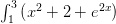.

Solution: We have

ORQ.24. Using integration, find the area of the triangular region whose sides have the equation y = 2x + 1, y = 3x + 1 and x = 4.

Solution: The equations of sides of triangle are

y = 2x + 1, …(i)

y = 3x + 1 …(ii)

and x = 4 …(iii)

The equation y = 2x + 1 meets x and y axes at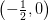and (0, 1). By joining these two points we obtain the graph of x + 2y = 2. Similarly, graphs of other equations are drawn.

Solving equation (i), (ii) and (iii) in pairs, we obtain the coordinates of vertices of ∆ABC are A(0, 1), B (4, 13) and C (4, 9).

Then, area of ∆ABC = Area (OLBAO) – Area (OLCAO)MathsSET-III

Section – AQ.1.Find the differenital equation representing the family of curves y = ae2x + 5, where a is an arbitrary constant.

Solution:

Given, y = ae2x + 5

On differentiating w.r.t. x, we getQ.2. If y = cos√3x, then find dy/dx

Solution:

Section – BQ.5. Show that the points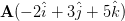,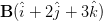and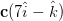are collinear.

Solution:Q.6. Find:

Solution:

Section – CQ.13. Solve for x: tan-1 + tan-1(x – 1) = tan-1(8/31).

Solution:Q.14. If x = aet (sin t + cost t) and y = aet (sin t – cos t), then prove that

dy/dx= x+y/x-y.

Solution:

dy/dx= x+y/x-y                  Hence proved.

ORQ.14. Differentiate xsinx + (sinx)cosx with respect to x.

Solution:Q.15. Find:

Solution:

Section – DQ.24.

Show that for the matrix A =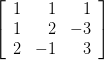, A3 – 6A2 + 5A + 111 = 0. Hence, find A -1.

Solution:

ORQ.24. Using matrix method, solve the following system of equations:

3x – 2y + 3z = 8

2x + y – z = 1

4x – 3y + 2z = 4

Solution:

The given equations are

3x – 2y + 3z = 8

2x + y – z = 1

4x – 3y + 2z = 4

These equations can be written in the form AX = B, whereQ.26. A bag contains 5 red and 4 black balls, a second bag contains 3 red and 6 black balls. One of the two bags is selected at random and two balls are drawn at random (without replacement) both of which are found to be red. Find the probability that the balls are drawn from the second bag.

Solution:

Let E1 be the event of choosing the bag I, E2 be the event of choosing the bag II and A be the event of drawing a red ball.

Then,